Technical Test SSC JE: Electrical Engineering (EE)- 10

# Technical Test SSC JE: Electrical Engineering (EE)- 10

Test Description

## 100 Questions MCQ Test Mock Test Series for SSC JE Electrical Engineering | Technical Test SSC JE: Electrical Engineering (EE)- 10

Technical Test SSC JE: Electrical Engineering (EE)- 10 for Electrical Engineering (EE) 2022 is part of Mock Test Series for SSC JE Electrical Engineering preparation. The Technical Test SSC JE: Electrical Engineering (EE)- 10 questions and answers have been prepared according to the Electrical Engineering (EE) exam syllabus.The Technical Test SSC JE: Electrical Engineering (EE)- 10 MCQs are made for Electrical Engineering (EE) 2022 Exam. Find important definitions, questions, notes, meanings, examples, exercises, MCQs and online tests for Technical Test SSC JE: Electrical Engineering (EE)- 10 below.
Solutions of Technical Test SSC JE: Electrical Engineering (EE)- 10 questions in English are available as part of our Mock Test Series for SSC JE Electrical Engineering for Electrical Engineering (EE) & Technical Test SSC JE: Electrical Engineering (EE)- 10 solutions in Hindi for Mock Test Series for SSC JE Electrical Engineering course. Download more important topics, notes, lectures and mock test series for Electrical Engineering (EE) Exam by signing up for free. Attempt Technical Test SSC JE: Electrical Engineering (EE)- 10 | 100 questions in 100 minutes | Mock test for Electrical Engineering (EE) preparation | Free important questions MCQ to study Mock Test Series for SSC JE Electrical Engineering for Electrical Engineering (EE) Exam | Download free PDF with solutions
 1 Crore+ students have signed up on EduRev. Have you?
Technical Test SSC JE: Electrical Engineering (EE)- 10 - Question 1

### The Req for the circuit shown in figure is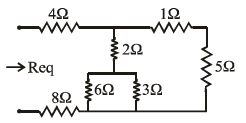Technical Test SSC JE: Electrical Engineering (EE)- 10 - Question 2

### Determine the motor current in a 440V crane which hoists a load of 50 KN at a uniform velocity of 0.5 m sec–1 if the efficiency is 80%

Detailed Solution for Technical Test SSC JE: Electrical Engineering (EE)- 10 - Question 2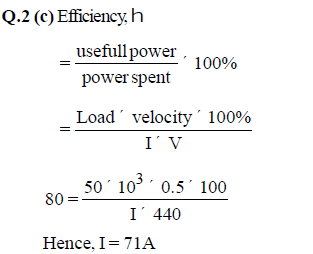Technical Test SSC JE: Electrical Engineering (EE)- 10 - Question 3

### If 220 V, 3A alternating current is to be converted into 5V, what is the current in thesecondary

Detailed Solution for Technical Test SSC JE: Electrical Engineering (EE)- 10 - Question 3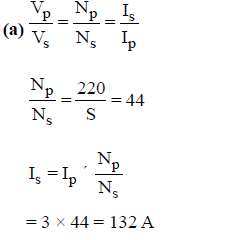Technical Test SSC JE: Electrical Engineering (EE)- 10 - Question 4

The DC resistance of conductor is less than AC resistance due to

Technical Test SSC JE: Electrical Engineering (EE)- 10 - Question 5

If the height of the transmission tower is increased

Technical Test SSC JE: Electrical Engineering (EE)- 10 - Question 6

On increasing the 'Q' of the coil

Detailed Solution for Technical Test SSC JE: Electrical Engineering (EE)- 10 - Question 6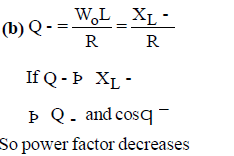Technical Test SSC JE: Electrical Engineering (EE)- 10 - Question 7

When a source is delivering maximum power to a load, the efficiency of the circuit

Technical Test SSC JE: Electrical Engineering (EE)- 10 - Question 8

An RLC resonant circuit has a resonance frequency of 1.5 MHz and bandwidth of 10
KHz. If C = 150 pf, then the effective resistance of the circuit will be

Detailed Solution for Technical Test SSC JE: Electrical Engineering (EE)- 10 - Question 8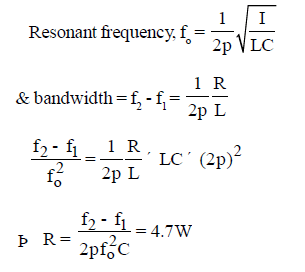Technical Test SSC JE: Electrical Engineering (EE)- 10 - Question 9

Bimetallic strip is

Technical Test SSC JE: Electrical Engineering (EE)- 10 - Question 10

A single phase full Bridge diode rectifier is supplied from 230V, 50 Hz source. The load consist of R =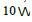then average value of output current is

Detailed Solution for Technical Test SSC JE: Electrical Engineering (EE)- 10 - Question 10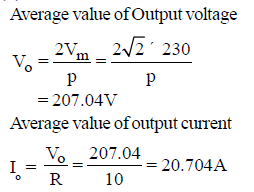Technical Test SSC JE: Electrical Engineering (EE)- 10 - Question 11

Horizontal hold control in a TV is a

Technical Test SSC JE: Electrical Engineering (EE)- 10 - Question 12

The efficiency of an incandescent lamp is

Technical Test SSC JE: Electrical Engineering (EE)- 10 - Question 13

What is the current through the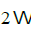resistance for the circuit as shown below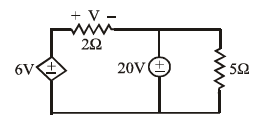Detailed Solution for Technical Test SSC JE: Electrical Engineering (EE)- 10 - Question 13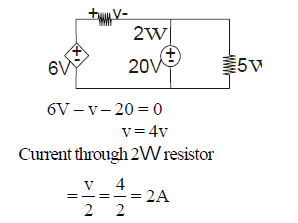Technical Test SSC JE: Electrical Engineering (EE)- 10 - Question 14

In RLC circuit, the current at resonance is

Technical Test SSC JE: Electrical Engineering (EE)- 10 - Question 15

A 3-f star connected balanced load consumes P watts of power from a 400V (line to line voltage) supply. If the same load is connected in delta across the same supply. What is the power consumption

Detailed Solution for Technical Test SSC JE: Electrical Engineering (EE)- 10 - Question 15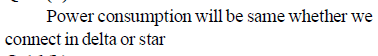Technical Test SSC JE: Electrical Engineering (EE)- 10 - Question 16

Two resistors of equal value are connected in series across the line B and C of a symmetrical 3-f 400V system. What is the magnitude of the voltage between the line A and the junction of the resistors

Detailed Solution for Technical Test SSC JE: Electrical Engineering (EE)- 10 - Question 16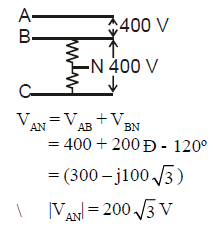Technical Test SSC JE: Electrical Engineering (EE)- 10 - Question 17

If load on a d.c. shunt motor is increased its speed is decreased due primarily to

Technical Test SSC JE: Electrical Engineering (EE)- 10 - Question 18

If the field circuit of a loaded shunt motor is suddenly opened

Technical Test SSC JE: Electrical Engineering (EE)- 10 - Question 19

Which of the following d.c. motor would be suitable for drives requiring high starting torquebut only fairly constant speed such as crushers

Technical Test SSC JE: Electrical Engineering (EE)- 10 - Question 20

Discharging action of points is utilised in

Technical Test SSC JE: Electrical Engineering (EE)- 10 - Question 21

What will happen if an ac series motor is run on a dc supply

Technical Test SSC JE: Electrical Engineering (EE)- 10 - Question 22

The current waveform as shown below is applied to in a pure resistor of 10W. What is the power disipated in the resistor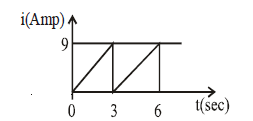Detailed Solution for Technical Test SSC JE: Electrical Engineering (EE)- 10 - Question 22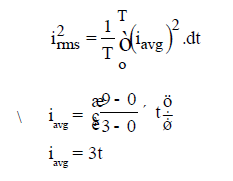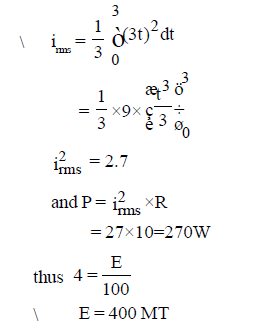Technical Test SSC JE: Electrical Engineering (EE)- 10 - Question 23

Which one of the following instruments is commonly used to measure primary current ofa transformer connected to mains

Technical Test SSC JE: Electrical Engineering (EE)- 10 - Question 24

The efficiency of dc generator is maximum when

Technical Test SSC JE: Electrical Engineering (EE)- 10 - Question 25

Magnetic lines of force coming from a magnet

Technical Test SSC JE: Electrical Engineering (EE)- 10 - Question 26

In case of frosted GLS lamps, frosting is done by

Technical Test SSC JE: Electrical Engineering (EE)- 10 - Question 27

In a motor starter, the electro mechanical contactor provides inherent protection against

Technical Test SSC JE: Electrical Engineering (EE)- 10 - Question 28

A good illumination will be, when the light is

Technical Test SSC JE: Electrical Engineering (EE)- 10 - Question 29

For the circuit as shown below, what is the value of I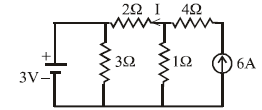Detailed Solution for Technical Test SSC JE: Electrical Engineering (EE)- 10 - Question 29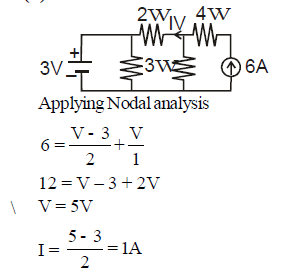Technical Test SSC JE: Electrical Engineering (EE)- 10 - Question 30

For a series RLC circuit energized with a sinusoidal voltage source of frequency 4 rad/sthe applied voltage lags the current by an angle of tan-12 degrees. Then the value of R for L=1Hand C = 0.05F is

Detailed Solution for Technical Test SSC JE: Electrical Engineering (EE)- 10 - Question 30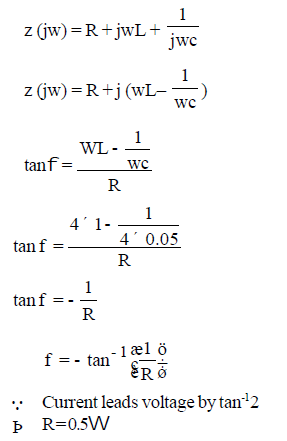Technical Test SSC JE: Electrical Engineering (EE)- 10 - Question 31

If the power dissipated in the circuit shown below is 8 W, then the value of E will be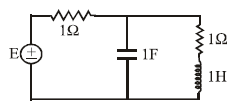Detailed Solution for Technical Test SSC JE: Electrical Engineering (EE)- 10 - Question 31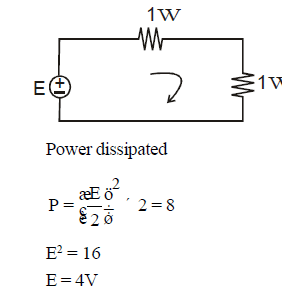Technical Test SSC JE: Electrical Engineering (EE)- 10 - Question 32

Decible scale is useful while measuring voltages covering

Technical Test SSC JE: Electrical Engineering (EE)- 10 - Question 33

For time and frequency, the working standard is

Technical Test SSC JE: Electrical Engineering (EE)- 10 - Question 34

Sensitivity of LVDT is mainly due to

Technical Test SSC JE: Electrical Engineering (EE)- 10 - Question 35

A d'Arsonval movement with internal resistance R=100 and full scale current of 1mA is to be
converted into (0-10)V range. What is the required resistance

Detailed Solution for Technical Test SSC JE: Electrical Engineering (EE)- 10 - Question 35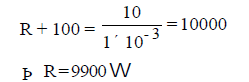Technical Test SSC JE: Electrical Engineering (EE)- 10 - Question 36

A triangular mmf wave is produced in the air gap of an electric machine, such a wave is produced by

Technical Test SSC JE: Electrical Engineering (EE)- 10 - Question 37

The induced emf of a dc machine running at 750 rpm is 220V. The percentage increase in field flux for generating an induced emf of 250V at 700 rpm would be

Detailed Solution for Technical Test SSC JE: Electrical Engineering (EE)- 10 - Question 37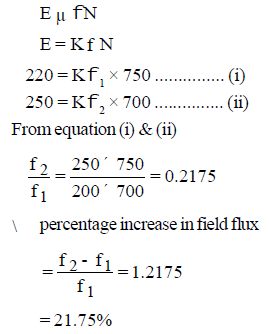Technical Test SSC JE: Electrical Engineering (EE)- 10 - Question 38

In dc machines, the demagnetising effect of armature reaction is due to

Technical Test SSC JE: Electrical Engineering (EE)- 10 - Question 39

If the fault current is 2000A, the relay setting is 50% and CT ratio is 400/5 the plug setting multiplier will be

Detailed Solution for Technical Test SSC JE: Electrical Engineering (EE)- 10 - Question 39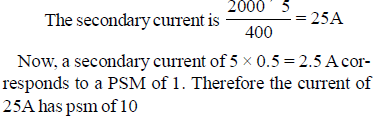Technical Test SSC JE: Electrical Engineering (EE)- 10 - Question 40

The average life of mercury vapour lamp is

Technical Test SSC JE: Electrical Engineering (EE)- 10 - Question 41

Which one of the following transducer is used to obtain the output positions in a position control system

Technical Test SSC JE: Electrical Engineering (EE)- 10 - Question 42

Semiconductor has a resistivity range from

Technical Test SSC JE: Electrical Engineering (EE)- 10 - Question 43

Secondary breakdown occurs in

Technical Test SSC JE: Electrical Engineering (EE)- 10 - Question 44

If PC and PSC represent core and full-load ohmic losses respectively, then maximum KVA
delivered to the load corresponding to maximum efficiency is equal to rated VA multiplied by

Technical Test SSC JE: Electrical Engineering (EE)- 10 - Question 45

What counters commutation in a d.c. machine

Technical Test SSC JE: Electrical Engineering (EE)- 10 - Question 46

Field control of a dc shunt motor gives

Technical Test SSC JE: Electrical Engineering (EE)- 10 - Question 47

Damper windings are provided on

Technical Test SSC JE: Electrical Engineering (EE)- 10 - Question 48

When is the ferranti effect on long overhead lines experienced

Technical Test SSC JE: Electrical Engineering (EE)- 10 - Question 49

Under-voltage relays are mainly used for

Technical Test SSC JE: Electrical Engineering (EE)- 10 - Question 50

Which is the main relay for protecting up to 90% of the transmission line-length in the forward direction

Technical Test SSC JE: Electrical Engineering (EE)- 10 - Question 51

To protect the power transformer (Y-Y with neutral grounded) against fault, what type of connections do the CTs have

Detailed Solution for Technical Test SSC JE: Electrical Engineering (EE)- 10 - Question 51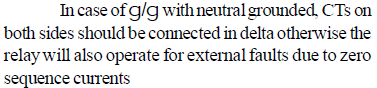Technical Test SSC JE: Electrical Engineering (EE)- 10 - Question 52

In a 220 KV system, the inductance and capacitance upto the circuit breaker location
are 25 mH and 0.025 mF respectively. The value of resistor required to be connected
across the breaker contacts which will give no transient oscillations is

Detailed Solution for Technical Test SSC JE: Electrical Engineering (EE)- 10 - Question 52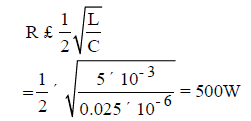Technical Test SSC JE: Electrical Engineering (EE)- 10 - Question 53

Electronic instruments for measurements of non electrical physical parameter use

Technical Test SSC JE: Electrical Engineering (EE)- 10 - Question 54

A DC voltmeter has sensitivity of 1000w /volt. When it measures half full scale in 100V range,the current through the voltmeter is

Detailed Solution for Technical Test SSC JE: Electrical Engineering (EE)- 10 - Question 54

The resistance of voltmeter is Rv = 1kΩ*1000v  =1mΩ

half scale the voltage across the voltmeter is 500V the current through the voltmeter Iv =

500/1mΩ = 5∗10−4A

=0.5mA

Technical Test SSC JE: Electrical Engineering (EE)- 10 - Question 55

Negative sequence relay is used in

Technical Test SSC JE: Electrical Engineering (EE)- 10 - Question 56

A transistor can provide

Technical Test SSC JE: Electrical Engineering (EE)- 10 - Question 57

Important electronic function which can be performed by a transistor is

Technical Test SSC JE: Electrical Engineering (EE)- 10 - Question 58

Tesla is same as

Technical Test SSC JE: Electrical Engineering (EE)- 10 - Question 59

The main advantage of temporary magnet is that we can

Technical Test SSC JE: Electrical Engineering (EE)- 10 - Question 60

Two holes are drilled in the disc on a diametern of energy meter to

Technical Test SSC JE: Electrical Engineering (EE)- 10 - Question 61

What is the typical use of an auto transformer

Technical Test SSC JE: Electrical Engineering (EE)- 10 - Question 62

In parallel operation of transformers, to reduce copper loss

Technical Test SSC JE: Electrical Engineering (EE)- 10 - Question 63

Fleming's left hand rule is applicable to

Technical Test SSC JE: Electrical Engineering (EE)- 10 - Question 64

Which of the following single phase motors is available with speed as low as one revolution per minute

Technical Test SSC JE: Electrical Engineering (EE)- 10 - Question 65

The daily energy produced in a thermal power station is 720 MWh at a load factor of 0.6.What is the maximum demand of the station

Detailed Solution for Technical Test SSC JE: Electrical Engineering (EE)- 10 - Question 65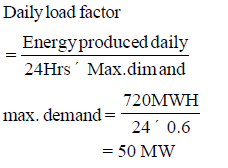Technical Test SSC JE: Electrical Engineering (EE)- 10 - Question 66

Which of the following power stations is mainly used to over peak load on the system

Technical Test SSC JE: Electrical Engineering (EE)- 10 - Question 67

For power transformers of large rating, the tapping are located in the middle portion of the winding to

Detailed Solution for Technical Test SSC JE: Electrical Engineering (EE)- 10 - Question 67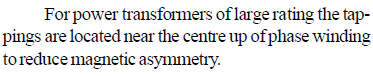Technical Test SSC JE: Electrical Engineering (EE)- 10 - Question 68

In an autotransformer, power is transferred through

Technical Test SSC JE: Electrical Engineering (EE)- 10 - Question 69

The applied voltage of a certain transformer isi ncreased by 75% while the frequency of the applied voltage is reduced by 25%. The maximum core flux density will

Detailed Solution for Technical Test SSC JE: Electrical Engineering (EE)- 10 - Question 69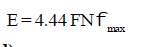Technical Test SSC JE: Electrical Engineering (EE)- 10 - Question 70

The advantage of the double squired cage induction motor over single cage rotor is that its

Detailed Solution for Technical Test SSC JE: Electrical Engineering (EE)- 10 - Question 70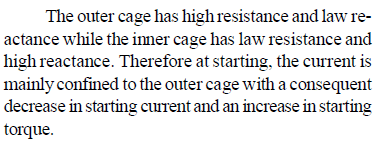Technical Test SSC JE: Electrical Engineering (EE)- 10 - Question 71

The injected emf in the rotor of induction motor must have

Technical Test SSC JE: Electrical Engineering (EE)- 10 - Question 72

In an induction motor, when the number of stator slots is equal to an integral multiple of rotor slots

Detailed Solution for Technical Test SSC JE: Electrical Engineering (EE)- 10 - Question 72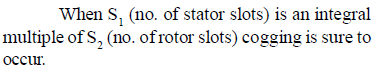Technical Test SSC JE: Electrical Engineering (EE)- 10 - Question 73

For maximum current during 'slip test 'synchronous machine, the armature mmf align salong

Technical Test SSC JE: Electrical Engineering (EE)- 10 - Question 74

Stepper motors are mostly used for

Technical Test SSC JE: Electrical Engineering (EE)- 10 - Question 75

Compared to breaking capacity of a circuit breaker its making capacity should be

Technical Test SSC JE: Electrical Engineering (EE)- 10 - Question 76

Resistance switching is normally employed in

Technical Test SSC JE: Electrical Engineering (EE)- 10 - Question 77

Silicon content in iron lamination is kept within 5% as it

Technical Test SSC JE: Electrical Engineering (EE)- 10 - Question 78

To minimize the error due to lead and contact resistances, low resistances used in electrical measurement work are provided with

Technical Test SSC JE: Electrical Engineering (EE)- 10 - Question 79

Hysteresis is the phenomenon in the magnetic circuit by which

Technical Test SSC JE: Electrical Engineering (EE)- 10 - Question 80

A wire placed on the top of a transmission line acts as

Technical Test SSC JE: Electrical Engineering (EE)- 10 - Question 81

An imperfect capacitor is represented by a capacitance C in parallel with a resistance R. The value of its dissipation factor tan d is

Technical Test SSC JE: Electrical Engineering (EE)- 10 - Question 82

Schering Bridge can be used to measure

Technical Test SSC JE: Electrical Engineering (EE)- 10 - Question 83

The bridge circuit shown in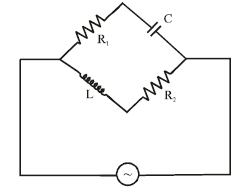Technical Test SSC JE: Electrical Engineering (EE)- 10 - Question 84

If the battery of the multimeter is weak, it will give

Technical Test SSC JE: Electrical Engineering (EE)- 10 - Question 85

Which of the multimeter is more sensitive and accurate

Technical Test SSC JE: Electrical Engineering (EE)- 10 - Question 86

An operating voltage of a particular multimeter is

Technical Test SSC JE: Electrical Engineering (EE)- 10 - Question 87

Multimeter can measure

Technical Test SSC JE: Electrical Engineering (EE)- 10 - Question 88

As per I.E. rules the insulation resistance between conductor and Earth should not beless than

Technical Test SSC JE: Electrical Engineering (EE)- 10 - Question 89

The insulation resistance of a installation between conductor to conductor should not be less than

Technical Test SSC JE: Electrical Engineering (EE)- 10 - Question 90

Which of the megger is better for testing installation

Technical Test SSC JE: Electrical Engineering (EE)- 10 - Question 91

Speed of the megger is kept at

Technical Test SSC JE: Electrical Engineering (EE)- 10 - Question 92

If the megger terminals are connected to 230 V supply, megger will

Technical Test SSC JE: Electrical Engineering (EE)- 10 - Question 93

The meter constant of energy meter is given by

Technical Test SSC JE: Electrical Engineering (EE)- 10 - Question 94

How will you identify wheather the meter 28 MI type of M.C. type from

Technical Test SSC JE: Electrical Engineering (EE)- 10 - Question 95

Chemical effect is used for the operation of

Technical Test SSC JE: Electrical Engineering (EE)- 10 - Question 96

A R-L circuit has 6 ohms resistance and 8 ohms inductive reactance. Its impedance willbe

Technical Test SSC JE: Electrical Engineering (EE)- 10 - Question 97

The unit of m.m.f. is

Technical Test SSC JE: Electrical Engineering (EE)- 10 - Question 98

An air condenser with capacitance 0.001 mF is connected to a d.c. voltage of 200 volts.The energy stored in the condenser will be

Technical Test SSC JE: Electrical Engineering (EE)- 10 - Question 99

One coulomb is equal to

Technical Test SSC JE: Electrical Engineering (EE)- 10 - Question 100

In metallic arc welding, the supply voltage may be

## Mock Test Series for SSC JE Electrical Engineering

2 videos|1 docs|55 tests
 Use Code STAYHOME200 and get INR 200 additional OFF Use Coupon Code
Information about Technical Test SSC JE: Electrical Engineering (EE)- 10 Page
In this test you can find the Exam questions for Technical Test SSC JE: Electrical Engineering (EE)- 10 solved & explained in the simplest way possible. Besides giving Questions and answers for Technical Test SSC JE: Electrical Engineering (EE)- 10, EduRev gives you an ample number of Online tests for practice

## Mock Test Series for SSC JE Electrical Engineering

2 videos|1 docs|55 tests